# ML Aggarwal Solutions Class 10 Maths Chapter 15 Circles

1. Using the given information, find the value of x in each of the following figures:Solution:
We have given that:
(i)∠ADB and ∠ACB are in the same segment.

Now in
∠DAB + X + ∠ADB = 180°
= 42°  + x + 50°  = 180°
= 92°  + x = 180°
x = 180° – 92°
x = 88°
(ii) In the given figure
we have
= 32°  + 45°  + x = 180°
= 77°  + x = 180°
x = 103°
(iii) From the given number
we have
Because angles in the same segment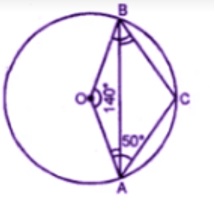∠BCD = 20°
∠CEA = 90°
∠CED = 90°
Now in triangle CED,
∠CED + ∠BCD + ∠CDE = 180°
90°  + 20°  + x = 180°
= 110°  + x = 180°
x = 180°  – 110°
x = 70°
(iv) In
∆ABC
∠ABC + ∠ABC + ∠BAC = 180°
(Because sum of a triangle)
69o + 31o + ∠BAC = 180°
∠BAC = 180°  – 100o
∠BAC = 80°
Since ∠BAC and ∠BAD are in the same
Segment.
(v) Given ∠CPB = 120°  ,∠ACP = 70°
To find, x° i,e., ∠PBD
Reflex ∠CPB = ∠BPO + ∠CPA
120°  = ∠BPD + ∠BPD
(BPD = CPA are vertically opposite ∠s)
2∠BPD = 120°  ∠PBD = 120°/2 = 60°
Also ∠ACP and PBD are in the same segment
∠PBD + ∠ACP = 70°
Now, In ∆PBD
∠PBD + ∠PDB + ∠BPD = 180°
(sum of all ∠s in a triangle)
70°  + x°  + 60°  = 180°
x = 180°  – 130°
x = 50°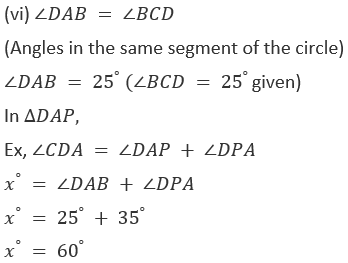2. If O is the center of the circle, find the value of x in each of the following figures (using the given information):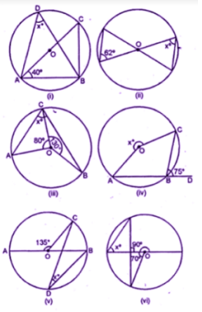Solution:
We have given that:
(Angles in the same segment of a circle)

∠ABC = x°
Now in ∆ABC
∠CAB + ∠ABC + ∠ACB = 180°
40°  + 90°  + x°  = 180°
(AC is the diameter)
130°  + x°  = 180°
x°  = 180°  – 130°  = 50°
(ii) ∠ACD = ∠ABD
(angles in the same segment)
∠ACD = x°
Now in triangle OAC,
OA = OC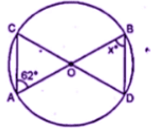∠ACO = ∠AOC
(opposite angles of equal sides)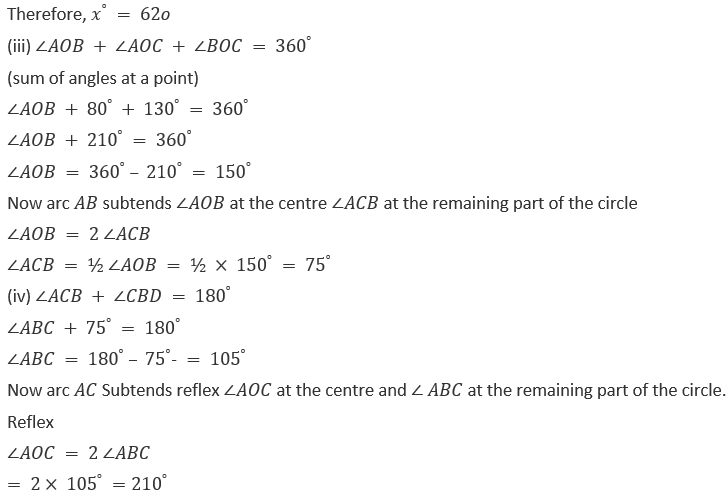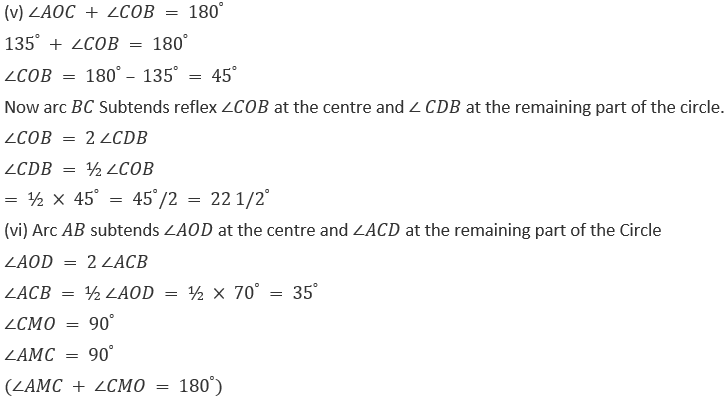3. (a) In the figure (i) given below, AD || BC.If ∠ACB = 35°. Find the measurement of ∠DBC.

(b) In the figure (ii) given below, it is given that O is the centre of the circle and ∠AOC = 130°. Find ∠ ABCSolution:
We have given that:
(a) Construction: Join AB
∠A = ∠C = 35° (Alt Angles)
∠ABC = 35°
(b) ∠AOC + reflex ∠AOC = 360°
130°  + Reflex ∠AOC = 360°
Reflex
∠AOC = 360° – 130° = 230°
Now arc BC Subtends reflex ∠AOC at the centre and ∠ ABC at the remaining part of the circle.
Reflex ∠AOC = 2 ∠ABC
∠ABC =1/2 reflex ∠AOC
= ½ × 230°  = 115°

4. a) In the figure (i) given below, calculate the values of x and y .
(b) In the figure (ii) given below, O is the centre of the circle. Calculate the values of x and y.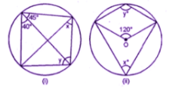Solution:
We have given that:∠B + ∠D = 180°
Y + 40°  + 45°  = 180°
(y + 85°  = 180°
Y = 180°  – 85°  = 95°
x°  = 40
(a) Arc ADC Subtends ∠AOC at the centre and
∠ ABC at the remaining part of the circle
∠AOC = 2 ∠ABC
x°= 60°
Again ABCD is a Cyclic quadrilateral
∠B + ∠D = 180°
60°  + yo = 180°
y = 180°  – 60°  = 120°

5. (a) In the figure (i) given below, M,A,B,N are points on a circle having centre O. AN and MB cut at Y. If ∠NYB = 50° and ∠YNB = 20°, find ∠MAN and the reflex angle MON.
(b) In the figure (ii) given below, O is the centre of the circle. If ∠AOB = 140° and ∠OAC = 50°, find
(i) ∠ACB
(ii) ∠OBC
(iii) ∠OAB
(iv) ∠CBA
Solution:
We have given that:
(a) ∠NYB = 50°,∠YNB = 20°.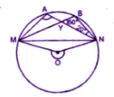In
∆YNB,
∠NYB + ∠YNB + ∠YBN = 180°
50°  + 20°  + ∠YBN = 180°
∠YBN + 70°  = 180°
∠YBN = 180°  – 70°  = 110°
But ∠MAN = ∠YBN
(Angles in the same segment)
∠MAN = 110°
Major arc MN subtend reflex ∠MON at the
Centre and ∠MAN at the remaining part of
the choice.
Reflex ∠MAN at the remaining part of the circle
Reflex ∠MON = 2 ∠MAN = 2 × 110 °=220°
(b) (i) ∠AOB + reflex ∠AOB = 360°
(Angles at the point)
140°  + reflex ∠AOB = 360°
Reflex ∠AOB = 360°  – 140°  = 220°
Now major arc AB subtends ∠AOB + ∠OBC = 360°50°  + 110°  + 140°  + ∠OBC = 360°
300°  + ∠OBC = 360°
∠300°  + ∠OBC = 360°
∠OBC = 360°   – 300°
∠OBC = 60°
∠OAC + ∠ACB + ∠AOB + ∠OBC = 360°
50o + 110°  + 140°  + ∠OBC = 360°
300°  + ∠OBC = 360°
∠OBC = 360°  – 300°
∠OBC =60°
(iii) in ∆OAB,
OA = OB
∠OAB + ∠OBA = 180°
2 ∠OAB = 180°  – 140°  = 40°
∠OAB = 40°/2 = 20°
But ∠OBC = 60°
∠CBA = ∠OBC – ∠OBA
= 60°  – 20°  = 40°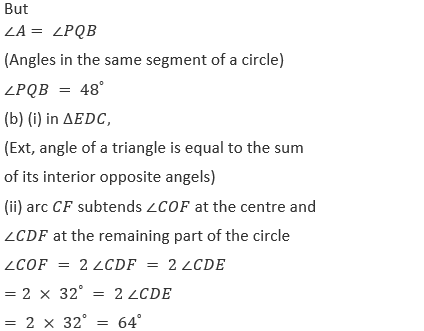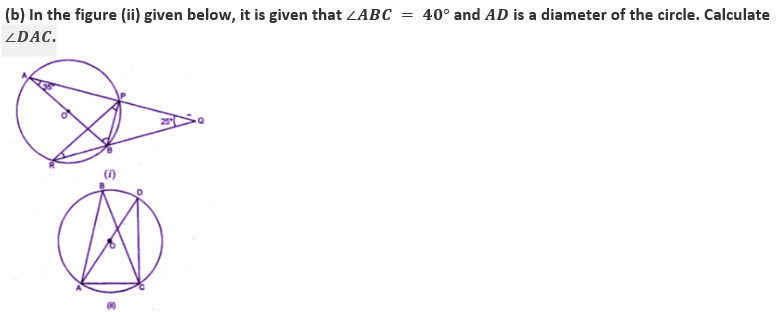Solution:
We have given that:
(a) (i) ∠PRB = ∠BAP

(Angles in the same segment of the circle)

∴∠PRB = 35° (∵ ∠BAP = 35° given)

8. (a) In the figure given below, P and Q are centers of two circles intersecting at B and C.ACD is a straight line. Calculate the numerical value of x.(b) In the figure given below, O is the circumcenter of triangle ABC in which AC = BC. Given that ∠ACB = 56°, calculate
(i)∠CAB
(ii)∠OAC
Solution:
We have given that:

(a) Arc AB subtends ∠APB at the center and ∠ACB at the remaining part of the circle
∠ACB = ½ ∠APB = ½ × 130°  = 65°
But ∠ACB + ∠BCD = 180°
(Linear Pair)
65°  + ∠BCD = 180°
∠BCD = 180° – 65° = 115°
Major arc BD subtends reflex ∠BQD at the Centre and ∠BCD at the remaining part of the circle reflex BQD =2 ∠BCD
=2 × 115°  = 230°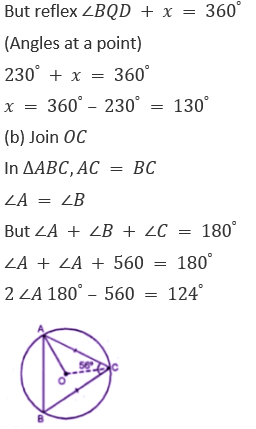∠A = 124/2 = 62° or ∠CAB = 62°
OC is the radius of the circle
OC bisects ∠ACB
∠OCA = ½ ∠ACB = ½ × 56°  = 28°
Now in ∆OAC
OA = OC
∠OAC = ∠OCA = 28°

Exercise 15.2

1. If O is the center of the circle, find the value of x in each of the following figures (using the given information)Solution:
We have given that:
From the figure
(i) ABCD is a cyclic quadrilateralNow arc BD subtends ∠BOD at the center
And ∠BAD at the remaining part of the circle.
∠BOD = 2 ∠BAD = 2 x
2 x = 150°  (x = 75°
(ii) ∠BCD + ∠DCE = 180°
(Linear pair)∠BCD + 80°  = 180°
∠BCD = 180°  – 80°  = 100°
Arc BAD subtends reflex ∠BOD at the
Centre and ∠BCD at the remaining part of the circle
Reflex ∠BOD = 2 ∠BCD
X°  = 2 × 100°  = 200°
(iii) In ∆ACB,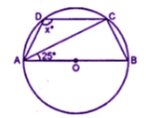∠CAB + ∠ABC + ∠ACB = 180°
(Angles of a triangle)
But
∠ACB = 90°
((Angles of a semicircle)
25°+ 90°  + ∠ABC = 180°
=115°  + ∠ABC = 180°
∠ABC = 180°  – 115°=65°
(Opposite angles of a cyclic quadrilateral)
65°  + x°  =180°
x°  = 180° -65°  = 115°

2. (a) In the figure (i) given below, O is the center of the circle. If ∠AOC = 150°, find (i) ∠ABC (ii) ∠ADC (b) In the figure (i) given below, AC is a diameter of the given circle and ∠BCD = 75°. Calculate the size of (i) ∠ABC (ii) ∠EAF.Solution:
(a) We have given that:
∠AOC = 150° and AD = CD
We know that an angle subtends by an arc of a circle
at the center is twice the angle subtended by the same arc
at any point on the remaining part of the circle.
(i) ∠AOC = 2 × ∠ABC
∠ABC = ∠AOC/2 = 150°/2 = 75°
(ii) From the figure, ABCD is a cyclic quadrilateral
(Sum of opposite angels in a cyclic quadrilateral
Is 180°)
(b) (i) AC is the diameter of the circle
∠ABC = 90° (Angle in a semi-circle)
(ii) ABCD is a cyclic quadrilateral
(∠BCD = 75°
∠BAD = 180°  -75°  = 105°
(Vertically opposite angles)
∠EAF = 105°

3. (a) In the figure, (i) given below, if ∠DBC = 58° and BD is a diameter of the circle, calculate:
(i) ∠BDC (ii) ∠BEC (iii) ∠BAC(b) In the figure (if) given below, AB is parallel to DC, ∠BCE = 80° and ∠BAC = 25°. Find: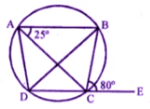Solution:
We have given that:
(a) ∠DBC = 58°
BD is diameter
∠DCB = 90° (Angle in semi-circle)
(i) In ∆BDC
∠BDC + ∠DCB + ∠CBD = 180°
∠BDC = 180°- 90° – 58° = 32°(ii) BEC = 180°  – 32°  = 148°
(iii) ∠BAC = ∠BDC = 32°
(Angles in same segment)
(b) in the figure, AB ∥DC
∠BCE = 80° and ∠BAC = 25°
ABCD is a cyclic Quadrilateral and DC is
Production to E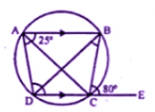(i) Ext, ∠BCE = interior ∠A
∠CAD = 80°  –25°  = 55°
(Alternate angels)
∠CBD = 55°
(iii) ∠BAC = ∠BDC
(Angles in the same segments)
∠BDC = 25°
(∠BAC = 25°
Now AB ∥ DC and BD is the transversal
∠BDC = ∠ABD
∠ABD = 25°
∠ABC = ∠ABD + ∠CBD = 25°  + 55°  = 80°
But ∠ABC + ∠ADC = 180°
(opposite angles of a cyclic quadrilateral)
∠ADC = 180°  – 80°  = 100°

4. (a) In the figure given below, ABCD is a cyclic quadrilateral. If ∠ADC = 80° and ∠ACD = 52°, find the values of ∠ABC and ∠CBD.(b) In the figure given below, O is the center of the circle. ∠AOE =150°,∠DAO = 51°. Calculate the sizes of ∠BEC and ∠EBC.
Solution:
We have given that:
(a) In the given figure, ABCD is a cyclic quadrilateral
∠ADC = 80° and ∠ACD = 52°
To find the measure of ∠ABC and ∠CBD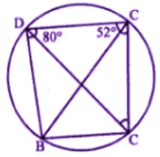(Sum of opposite angles =180°)
∠ABC + 80°  = 180°
∠AOE = 150°,∠DAO = 51°
To find ∠BEC and ∠EBC
Ext. ∠BEC = ∠DAB = 51°
∠ AOE = 150°
Ref ∠AOE = 360°  – 150°  = 51°
∠AOE = 150°
Ref ∠AOE = 360°  – 150°  = 210°
Now arc ABE subtends ∠AOE at the Centre
And ∠ADE at the remaining part of the circle.
∠ADE = ½ ref ∠AOE = ½ × 210°  = 105°
But Ext ∠EBC = ∠ADE = 105°
Hence ∠BEC = 51° and ∠EBC = 105°

5. (a) In the figure (i) given below, ABCD is a parallelogram. A circle passes through A and D and cuts AB at E and DC at F. Given that ∠BEF = 80°, find ∠ABC.
(b) In the figure (ii) given below, ABCD is a cyclic trapezium in which AD is parallel to BC and ∠B = 70°, find:
Solution:
We have given that:
ABCD is a parallelogram
∠B = ∠D = ∠ADF = 80°
or ∠ABC = 80°

(i) ∠B + ∠A = 180°
⇒ 70° + ∠A = 180°
⇒ ∠A = 180° – 70° = 110°
(ii) ABCD is a cyclic quadrilateral
∠A + ∠C = 180°
⇒ 110° + ∠C = 180°
⇒ ∠C = 180° – 110° = 70°
∠BCD = 70°

6. (a) In the figure given below, O is the center of the circle. If ∠BAD = 30°, find the values of p, q and r.(a) In the figure given below, two circles intersect at points P and Q. If ∠A = 80° and ∠D = 84°, calculate
(i) ∠QBC
(ii) ∠BCP
Solution:
We have given that:
(i) ABCD is a cyclic quadrilateral∠A + ∠C = 180°
30°  + p = 180°
P=180°  – 30°  = 150°
(ii) Arc BD subtends ∠BOD at the center
And ∠BAD at the remaining part of the circle
q = 2 × 30°  = 60°
∠BAD = ∠BED are in the same segment of the circle
30°  = r
r = 30°
Join PQ∠A + ∠QPD = 180°

7. (a) In the figure given below, PQ is a diameter. Chord SR is parallel to PQ.Given ∠PQR = 58°, calculate (i) ∠RPQ (ii) ∠STP
(T is a point on the minor arc SP)(b) In the figure given below, if ∠ACE = 43° and ∠CAF = 62°, find the values of a,b and c .
Solution:
(a) In ∆PQR,
∠PRQ = 90° (Angle in a semi-circle) and ∠PQR = 58°
∠RPQ = 90° – ∠PQR = 90° – 58° = 32°
SR || PQ (given)
∠SRP = ∠RPQ = 32° (Alternate angles)
Now PRST is a cyclic quadrilateral,
∠STP + ∠SRP = 180°
∠STP = 180°  – 32°  = 148°
(b) In the given figure,
∠ACE 〖43〗^° and ∠CAF = 62°
Now, in ∆AEC
∠ACE + ∠CAE + ∠AEC = 180°
43°  + 62°  + ∠AEC = 180°
105°  + ∠AEC = 180°
∠AEC = 180°   – 1050 = 75°
But ∠ABD + ∠AED = 180°
(sum of opposite angles of acyclic quadrilateral)
and ∠AED = ∠AEC
a + 75°  = 180°
a = 180°  – 75°  – 105°
but ∠EDF = ∠BAE
(Angles in the alternate segment)

8. (a) In the figure (i) given below, AB is a diameter of the circle. If ∠ADC = 120°, find ∠CAB.
(b) In the figure (ii) given below, sides AB and DC of a cyclic quadrilateral ABCD are produced to meet at E, the sides AD and BC are produced to meet at F. If x∶ y∶ z = 3∶ 4∶ 5, find the values of x,y and z.Solution:
(a) Construction: Join BC, and AC thenNow in ∆DCF
Ext. ∠2 = x + z
and in ∆CBE
Ext. ∠1 = x + y
x + y + x + z = ∠1 + ∠2
2 x + y + z = 180°
But x∶ y∶ z = 3∶ 4∶ 5
x/y = ¾ (y = 4/3 x)
x/z = 3/5 (z = 5/3 x.

Exercise 15.3

1. Find the length of the tangent drawn to a circle of radius 3cm, from a point distnt 5cm from the center.

Solution:In a circle with center O and radius 3cm and p is at a distance of 5cm.
That is OT = 3 cm,OP = 5 cm
OT is the radius of the circle
OT ⊥ PT
Now in right ∆ OTP, by Pythagoras axiom,
OP2  = OT2  + PT2
(5)2  = (3)2  + PT2
PT2   = (5)2  – (3)2  = 25 – 9 = 16 = (4)2
PT = 4 cm.
Hence, the given values PT = 4 cm are not roots of the equation.

2. A point P is at a distance 13 cm from the center C of a circle and PT is a tangent to the given circle. If PT = 12 cm, find the radius of the circle.
Solution:
CP = 13 cm and tangent PT = 12 cm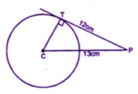CT is the radius and TP is the tangent
CT is perpendicular TP
Now in right angled triangle CPT,
CP2  = CT2  + PT2 [using Pythagoras axiom]
(13)2  = (CT)2  + (12)2
169 = (CT)2  + 144
(CT)2  = 169 -144 =25 = (5)2
CT = 5 cm.
Hence the radius of the circle is 5cm

3. The tangent to a circle of radius 6 cm from an external point P, is of length 8 cm. Calculate the distance of P from the nearest point of the circle.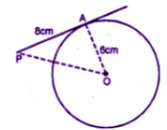Solution:
Radius of the circle = 6 cm

and length of tangent = 8 cm

Let OP be the distance

i.e. OA = 6 cm,AP = 8 cm .

OA ⊥ AP
Now In right ∆OAP,
OP2  = OA2  + AP2
(By Pythagoras axiom)
= (6)2+ (8)2
=36 + 64
= 100
= (10)2
OP = 10 cm.
Hence, the given values OP = 10 cm are not roots of the equation.

4. Two concentric circles are of the radii 13 cm and 5 cm. Find the length of the chord of the outer circle which touches the inner circle.

We have given that:

Solution:
Two concentric circles with center O
OP and OB are the radii of the circles respectively, then
OP = 5 cm,OB = 13 cm.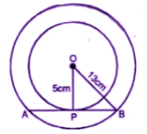Ab is the chord of outer circle which touches the inner circle at P.
OP is the radius and APB is the tangent to the inner circle.
In the right angled triangle OPB, by Pythagoras axiom,
OB2  = OP2  + PB2
132  = 52  + PB2
169 = 25 + PB2
PB2   = 169 – 25
= 144
PB = 12 cm
But P is the mid-point of AB.
AB = 2PB
= 24 cm

5. Two circles of radii 5 cm and 2-8 cm touch each other. Find the distance between their centers if they touch :
(i) externally
(ii) internally.
Solution:
Radii of the circles are 5 cm and 2.8 cm.
i.e. OP = 5 cm and CP = 2.8 cm.(i) When the circles touch externally, then the distance between their centers = OC = 5 + 2.8
= 7.8 cm.
(ii) When the circles touch internally, then the distance between their centers = OC = 5.0 – 2.8
= 2.2 cm

6. (a) In figure (i) given below, triangle ABC is circumscribed, find x.
(b) In figure (ii) given below, quadrilateral ABCD is circumscribed, find x.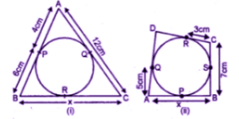Solution:
(a) From A,AP and AQ are the tangents to the circle
∴ AQ = AP = 4cm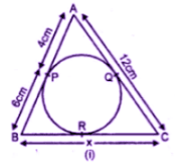But AC =12 cm
CQ = 12 – 4 = 8 cm.
From B,BP and BR are the tangents to the circle
BR = BP = 6 cm.
Similarly, from C,
CQ and CR the tangents
CR = CQ = 8 cm

x = BC = BR + CR = 6 cm + 8 cm = 14 cm
(b) From C,CR and CS are the tangents to the circle.CS = CR = 3 cm.
But BC = 7 cm.
BS = BC – CS = 7 – 3 =4 cm.
Now from B,BP and BS are the tangents to the circle.
BP = BS = 4 cm
From A,AP and AQ are the tangents to the circle.
AP = AQ = 5cm
x = AB = AP + BP = 5 + 4
= 9 cm

7. (a) In figure (i) given below, quadrilateral ABCD is circumscribed; find the perimeter of quadrilateral ABCD.
(b) In figure (ii) given below, quadrilateral ABCD is circumscribed and AD ⊥ DC ; find x if radius of incircle is 10 cm.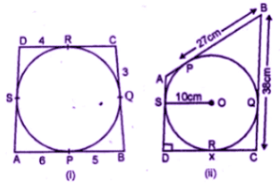Solution:
(a) From A,AP and AS are the tangents to the circle
∴AS = AP = 6
From B,BP and BQ are the tangents
∴BQ = BP = 5
From C,CQ and CR are the tangents
CR = CQ
From D,DS and DR are the tangents
DS = DR = 4
Therefore, perimeter of the quadrilateral ABCD
= 6 + 5 + 5 + 3 + 3 + 4 + 4 + 6
= 36 cm
(b) in the circle with center O, radius OS = 10 cm
PB = 27 cm,BC = 38 cm
SD = OS = 10 cm.Now from D,DR and DS are the tangents
To the circle
DR = DS = 10 cm
From B,BP and BQ are tangents to the circle.
BQ = BP = 27 cm.
CQ = CB – BQ = 38 – 27 = 11 cm.
Now from C,CQ and CR are the tangents to the circle
CR = CQ = 11 cm.
DC = x =DR + CR
= 10 + 11 = 21 cm

8. (a) In the figure (i) given below, O is the center of the circle and AB is a tangent at B. If AB = 15 cm and AC = 7.5 cm, find the radius of the circle.
(b) In the figure (ii) given below, from an external point P, tangents PA and PB are drawn to a circle. CE is a tangent to the circle at D. If AP = 15 cm, find the perimeter of the triangle PEC.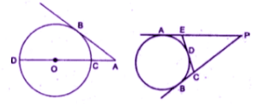Solution:
(i) Join OB
∠OBA = 90°
(Radius through the point of contact is perpendicular to the tangent)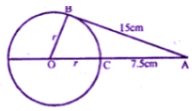OB2  = OA2  – AB2
r2  = (r + 7.5) 2– 152
r2  = r2  + 56.25 + 15r – 225
15r = 168.75
r = 11.25
Hence, radius of the circles = 11.25 cm
(ii) In the figure, PA and PB are the tangents
Drawn from P to the circle.CE is tangent at D
AP = 15 cm
PA and PB are tangents to the circle
AP = BP = 15 cm
Similarly EA and ED are tangents
EA = ED
Similarly BC = CD
Now perimeter of triangle PEC,
= PE + EC + PC
= PE + ED + CD + PC
PE + EA + CB + PC
(ED = EA and CB = CD)
=AP + PB = 15 + 15
= 30 cm.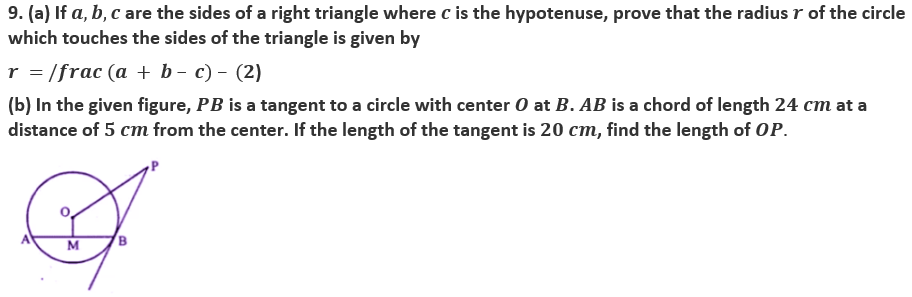Solution:
(a) Let the circle touch the sides BC,CA and AB of the right triangle ABC at points D,E and F respectively,

where BC = a,CA = b

and AB = c (as showing in the given figure).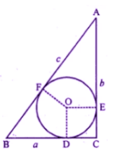As the lengths of tangents drawn from an
External point to a circle are equal
AE = AF,BD = BF and CD = DE
OD ⊥ BC and OE ⊥ CA
OD ⊥ BC and OE ⊥ CA
ODCE is a square of side r
DC = CE = r
AF = AE = AC – EC = b – r and
BF = BD = BC – DC = a – r
Now, AB = AF + BF
C = (b – r) + (a – r)
2r = a + b – c
r = a + b – c/2
OP2  = 400 + 169
OP = a-√569 cm
Hence, the given values OP = a-√569 cm are not roots of the equation.

10. Three circles of radii 2 cm,3 cm and 4 cm touch each other externally. Find the perimeter of the triangle obtained on joining the centers of these circles.
Solution:
We have given that:
Three circles with centers A,B and C touch each other externally at P,Q and R respectively and the radii of these circles are
2 cm,3 cm and 4 cm.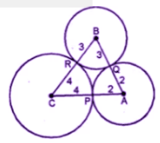By joining the centers of triangle ABC formed in which,
AB = 2 + 3 = 5 cm
BC = 3 + 4 = 7 cm
CA = 4 + 2 = 6 cm
Therefore, perimeter of the triangle ABC = AB + BC + CA
= 5 + 7 + 6
= 18 cm

Chapter Test

1. (a) In the figure (i) given below, triangle ABC is equilateral. Find ∠BDC and ∠BEC. (b) In the figure (ii) given below, AB is a diameter of a circle with center O.OD is perpendicular to AB and C is a point on the arc DB. Find ∠BAD and ∠ACD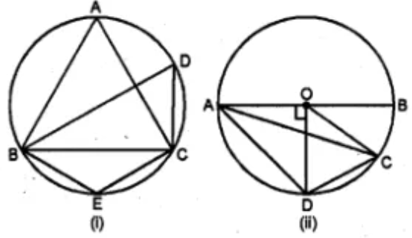Solution:
We have given that:
(a) triangle ABC is an equilateral triangleEach angle = 60°
∠A = 60°
But ∠A = ∠D
(Angles in the same segment)
∠D = 60°
Now ABEC is a cyclic quadrilateral,
∠A = ∠E = 180°
60°  + ∠E = 180°
60°  + ∠E = 180°  (∠E = 180°  – 60°
∠E = 120°
Hence ∠BDC = 60° and ∠BEC = 120°
1.AB is diameter of circle with centre O.
OD ⊥ AB and C is a point on arc DB.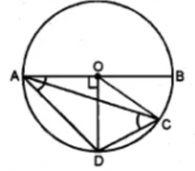In ∆AOD,∠AOD = 90°
OA = OD (raddi of the semi – circle)
But ∠OAD + ∠ODA = 90°
(ii) Arc AD subtends ∠AOD at the centre and
∠ACD at the remaining part of the circle
∠AOD = 2 ∠ACD
90°  = 2 ∠ACD (OD ⊥ AB)
∠ACD = 90°/2 = 45°Solution:
We have given that:
(a) Join DB,CA and CB. ∠ADC = 118° (given) and ∠ADB = 90°
(Angles in a semi-circle)
= 118°  – 90°  = 28°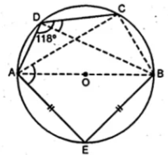118°  + ∠ABC = 180°
∠ABC = 180°  – 118°  = 62°
But in ∆AEB
∠AEB = 90°
(Angles in a semi-circle)
∠EAB = ∠ABE (AE = BE)
∠EAB + ∠ABE = 90°
∠EAB = 90°  × ½ = 45°
∠CBE = ∠ABC + ∠ABE
= 62°  + 45°  = 107°
But AEBD is a cyclic quadrilateral
∠CAE + ∠CBE = 180°
∠CAE + 107°  = 180°
∠CAE = 180°  – 107°  = 73°
(b) AB is the diameter of semi-circle ABCDE
With center O.AE = ED and ∠BCD = 140°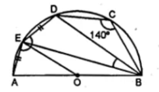(i) ∠BCD + ∠BED = 180°
140°  + ∠BED = 180°
∠BED = 180°  – 140°  = 40°
But ∠AED = 90°
(Angles in a semi circle)
∠AED = ∠AEB + ∠BED
= 90°  + 40°  = 130°
(ii) Now in cyclic quadrilateral AEDB
∠AED + ∠DBA = 180°
130°+ ∠DBA =180°
∠BDA = 180°  – 130°  = 50°
Chord AE = ED (given)
∠DBE = ∠EBA
But ∠DBE + ∠EBA = 50°
DBE + ∠DBE = 50°
2∠DBE = 50°
∠DBE = 25°  or ∠EBD = 25°
In ∆OEB,OE = OB
∠OEB = ∠EBO = ∠DBE
But these are ultimate angles
OE ∥ BD

3. a) In the figure (i) given below, O is the centre of the circle. Prove that ∠AOC = 2 (∠ACB + ∠BAC). (b) In the figure (ii) given below, O is the centre of the circle. Prove that x + y = zSolution :
We have given that:
(a) Given: O is the center of the circle. To Prove : ∠AOC = 2 (∠ACB + ∠BAC). Proof: In ∆ABC, ∠ACB + ∠BAC + ∠ABC = 180° (Angles of a triangle)
∠ABC = 180°  – (∠ACB + ∠BAC)….(i)
In the circle, arc AC subtends ∠AOC at
The center and ∠ABC at the remaining part of the circle.
Reflex ∠AOC = 2 ∠ABC …(ii)
Reflex AOC = 2 { (180°  – (ACB + BAC)}
But ∠AOC = 360°  – 2(∠ACB + ∠BAC)
But ∠AOC = 360°   – reflex ∠AOC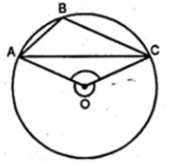=360 – 360°  – 2(∠ACB + ∠BAC)
= 360°  – 360°  + 2 (∠ACB + ∠BAC)
=2 (∠ACB + ∠BAC)
Hence ∠AOC = 2 (∠ACB + ∠BAC)
(b) Given : in the figure, O is the center of the circle
To Prove : × + y = z.Proof : Arc BC subtends ∠AOB at the center and ∠BEC at the remaining part of the circle.
∠BOC = 2 ∠BEC
But ∠BEC = ∠BDC
(Angles in the same segment)
∠BOC = ∠BEC + ∠BDC ……. (ii)
Similarly in ∆ABD
Ext. ∠BDC = x + ∠ABD
= x + ∠EBD ………….(iii)
Substituting the value of (ii) and (iii) in (i)
∠BOC = y – ∠EBD + x + ∠EBD = x + y
Z = x + y